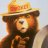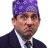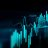# Convert custom indicator to scan

R

#### ReyHak

##### New member
Hi,
How do I change this so I an use it as an scan, for example so it can show me only stocks that it's between 50 and 100? I currently use this as an culumn in my watch-list.

def x = Average(volume, 50);
def v = volume;
plot z = Round(100 * ((v - x) / x), 1);

P

#### Pensar

##### Active member
VIP
@ReyHak Something like this?

Code:
``````def x = Average(volume, 50);
def v = volume;
def z = Round(100 * ((v - x) / x), 1);

plot scan = z > 50 and z < 100;``````
When scanning against all stocks (excluding OTC's) I'm currently getting 219 results.

R

#### ReyHak

##### New member
@Pensar this is perfect, thanks so much
Thanks for fixing my spelling as wellHow do I convert this ATR% chart study into a scanner watchlist column? Questions 4Convert encoded Mt4 to Thinkscript Questions 2Convert Chande Kroll Stop to ThinkorSwim Questions 2